# Irreducible component

In mathematics, and specifically in algebraic geometry, the concept of irreducible component is used to make formal the idea that a set such as defined by the equation

XY = 0

is the union of the two lines

X = 0

and

Y = 0.

Thus an algebraic set is irreducible if it is not the union of two proper algebraic subsets. It is a fundamental theorem of classical algebraic geometry that every algebraic set is the union of a finite number of irreducible algebraic subsets (varieties) and that this decomposition is unique if one removes those subsets that are contained in another one. The elements of this unique decomposition are called irreducible components.

This notion may be reformulated in topological terms, using Zariski topology, for which the closed sets are the subvarieties: an algebraic set is irreducible if it is not the union of two proper subsets that are closed for Zariski topology. This allows a generalization in topology, and, through it, to general schemes for which the above property of finite decomposition is not necessarily true.

## In topology

A topological space X is reducible if it can be written as a union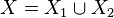$X = X_1 \cup X_2$ of two non-empty closed proper subsets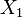$X_1$,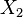$X_2$ of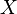$X$. A topological space is irreducible (or hyperconnected) if it is not reducible. Equivalently, all non empty open subsets of X are dense or any two nonempty open sets have nonempty intersection.

A subset F of a topological space X is called irreducible or reducible, if F considered as a topological space via the subspace topology has the corresponding property in the above sense. That is,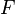$F$ is reducible if it can be written as a union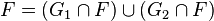$F = (G_1\cap F)\cup(G_2\cap F)$ where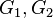$G_1,G_2$ are closed subsets of$X$, neither of which contains$F$.

An irreducible component of a topological space is a maximal irreducible subset. If a subset is irreducible, its closure is, so irreducible components are closed.

## In algebraic geometry

Every affine or projective algebraic set is defined as the set of the zeros of an ideal in a polynomial ring. In this case, the irreducible components are the varieties associated to the minimal primes over the ideal. This is this identification that allows to prove the uniqueness and the finiteness of the decomposition. This decomposition is strongly related with the primary decomposition of the ideal.

In general scheme theory, every scheme is the union of its irreducible components, but the number of components is not necessary finite. However, in most cases occurring in "practice", namely for all noetherian schemes, there are finitely many irreducible components.

## Examples

The irreducibility depends much on actual topology on some set. For example, possibly contradicting the intuition, the real numbers are reducible for their usual topology: they are the union of the two closed intervals ]-∞,0] and [0,+∞[.

The notion of irreducible component is fundamental in algebraic geometry and rarely considered outside this area of mathematics: consider the algebraic set

X := {(x, y) | xy = 0}.

It is subset of the plane. For the Zariski topology, its closed subsets are itself, the empty set, the singletons and the two lines defined by x = 0 and y = 0. It is thus reducible with these two lines as irreducible components.

This can also be read off the coordinate ring k[x, y]/(xy) (if the variety is defined over a field k), whose minimal prime ideals are (x) and (y).